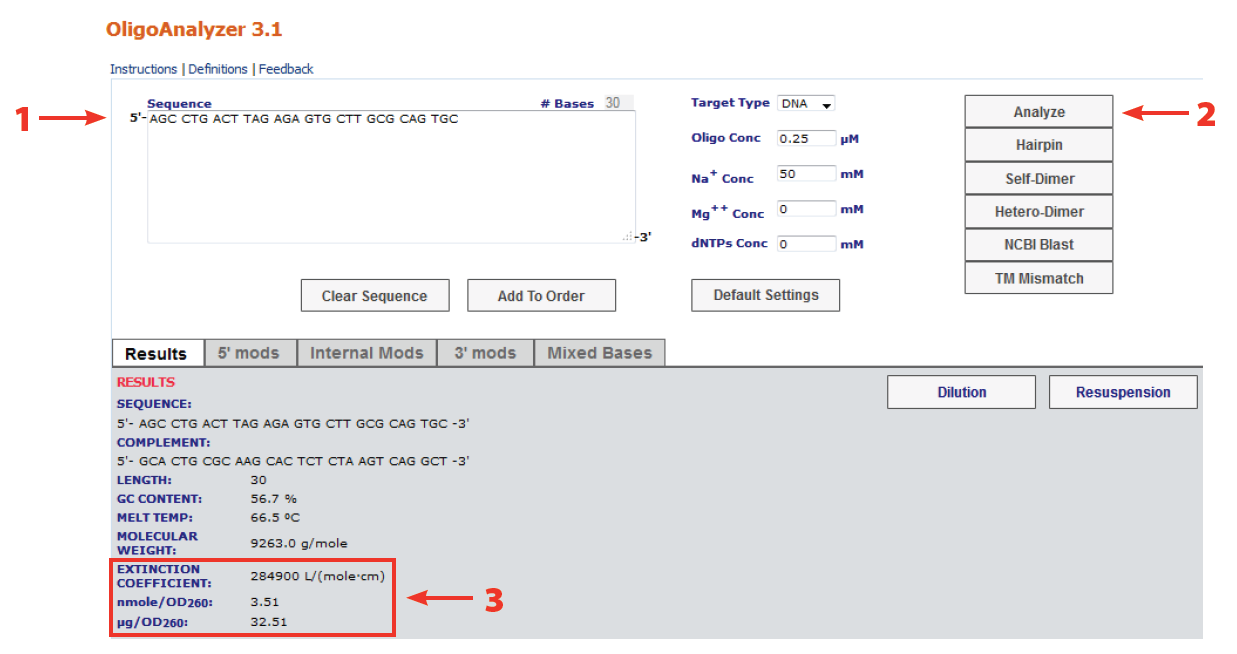# Oligo quantification—getting it rightDid you know that a supplier's yield readings can differ from what the researcher calculates after resuspension? Learn the importance of using the [right] molar extinction coefficient in calculations of oligonucleotide concentration.

We occasionally get calls from researchers who take issue with the yield readings we supply, saying they differ from what the researcher has calculated after resuspension. This article highlights the importance of using the [right] molar extinction coefficient in calculations of oligonucleotide concentration.

### Why include extinction coefficient in calculations?

Optical absorbance at 260 nm is routinely used to measure the concentration of nucleic acids present in a solution. Approximate conversion factors estimate that duplex DNA is about 50 μg/OD260, single-stranded RNA is approximately 40 μg/OD260, and single-stranded DNA is approximately 33 μg/OD260.

While these conversion factors may provide a reasonable estimate for long, essentially randomized sequences, they are less accurate for short oligonucleotides and repeating sequences. Conjugated double bonds are strong absorbers of light. They exhibit a property known as resonance, which causes them to absorb light of longer wavelengths (i.e., low energy). The molar absorptivity or extinction coefficient, and wavelength of maximum absorbance generally increase with increasing numbers of conjugated double bonds. Thus, the absorbance of each base is different, and base composition, sequence context, and sequence length all influence absorbance. In laymen’s terms, the extinction coefficient of a material describes the amount of light absorbed at a given concentration and distance travelled.

### By how much does sequence composition affect extinction coefficient?

Would you believe that the extinction coefficient for a sequence as short as 6 bases can vary just by arranging the bases in a different order? Additionally, base composition can lead to significant differences in the extinction coefficient. See Table 1 for examples.

Greatest accuracy is therefore achieved when the exact value of ε260 is calculated for each oligo. Further, it is necessary to take into account the presence of oligo modifications, such as fluorescent dyes, which may have significant absorbance at 260 nm.

For these reasons, IDT calculates the extinction coefficient for every oligo synthesized using a nearest neighbor method. This value is then used to measure the yield for each oligonucleotide produced.

### The nearest neighbor method

Nearest neighbor values for ε260 of dNTPs are:

We then use the formula [2,3],

Absorbance is calculated using the Beer-Lambert Law:

A = log(Io/I) = ε × c × p

where, A is absorbance; Io and I are, respectively, the intensities of incident and transmitted light; c is the molar concentration of an oligonucleotide (mole/L); p is the length of the light path through the sample (cm); and ε is the molecule molar extinction coefficient (L/mole•cm).

### Is there a program that can do that calculation for me?

The nearest neighbor calculation of extinction coefficient for any sequence can be calculated using the free IDT OligoAnalyzer® program (www.idtdna.com/SciTools), which will then go on to determine OD for that sequence (Figure 1).Figure 1. 3 Easy steps for determining oligonucleotide extinction coefficient and concentration. The OligoAnalyzer® program can be accessed at www.idtdna.com/SciTools. Step 1: Paste or type in the oligo sequence of interest. Step 2: Click on the Analyze button. Step 3: Read out your results.